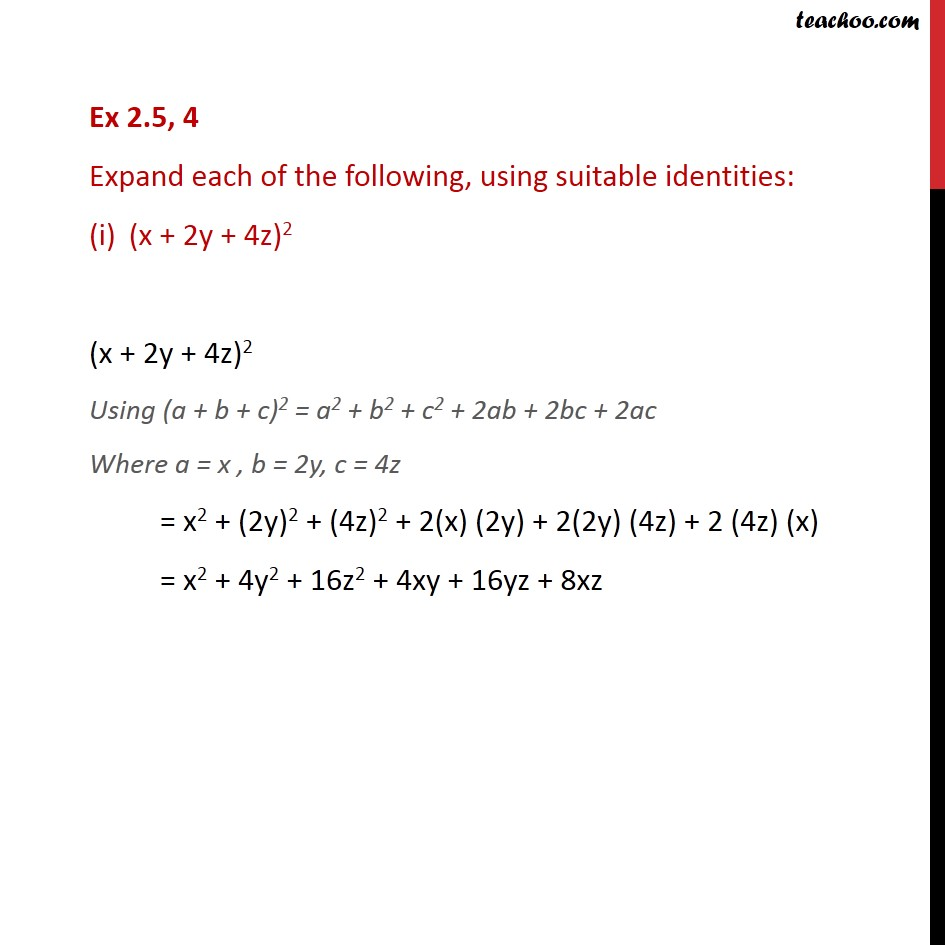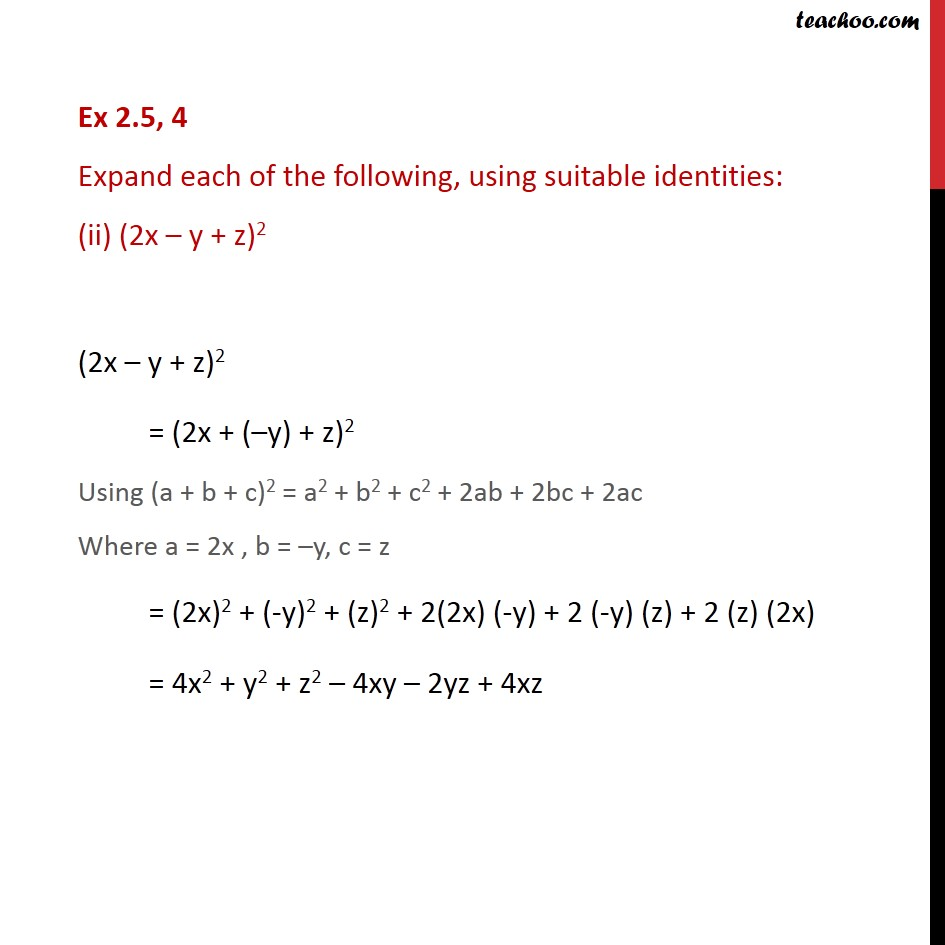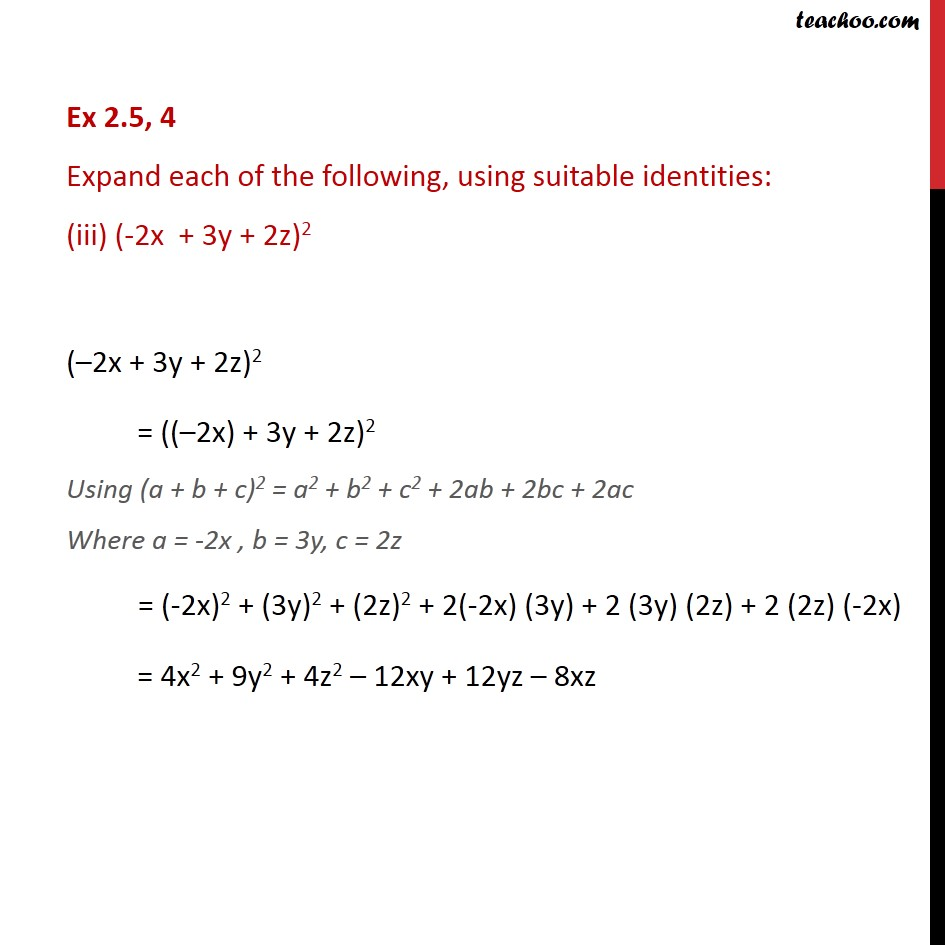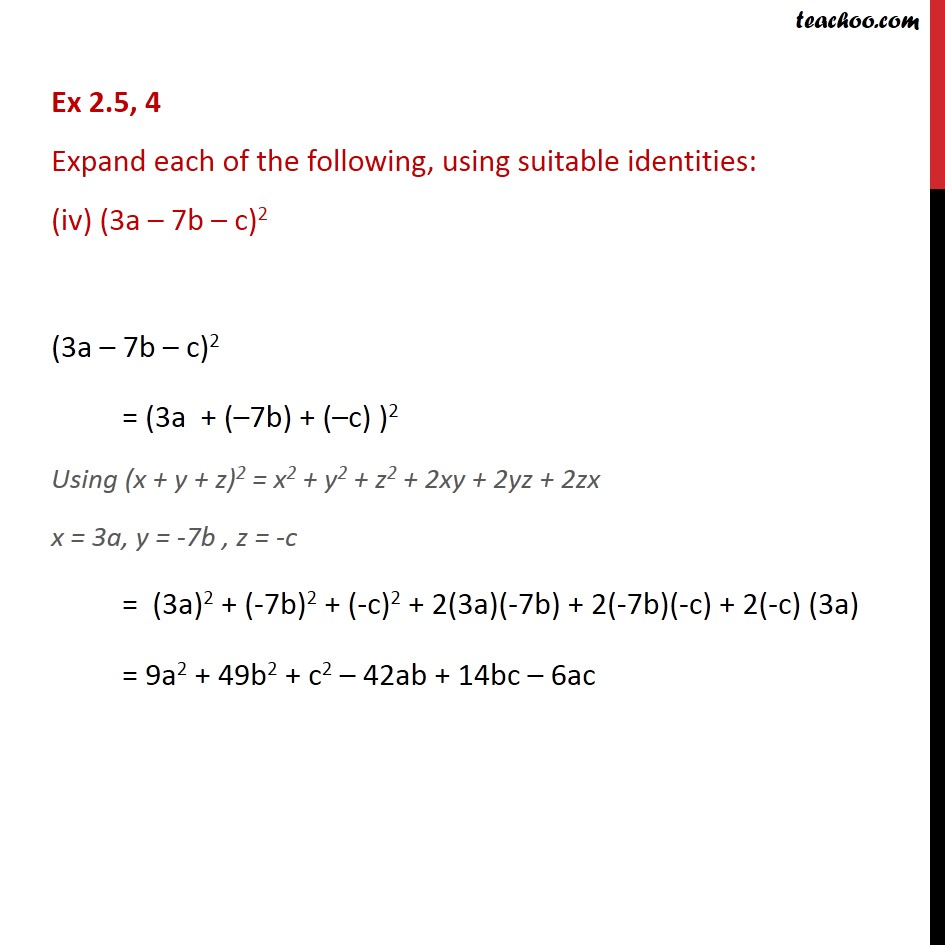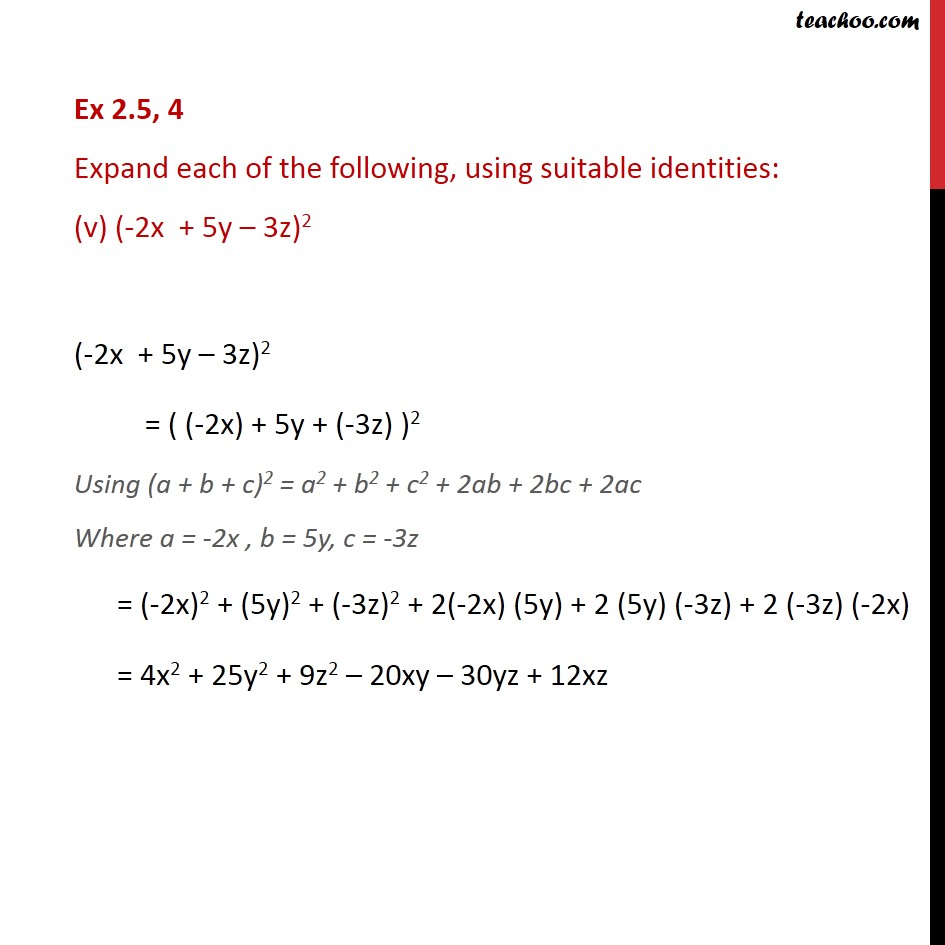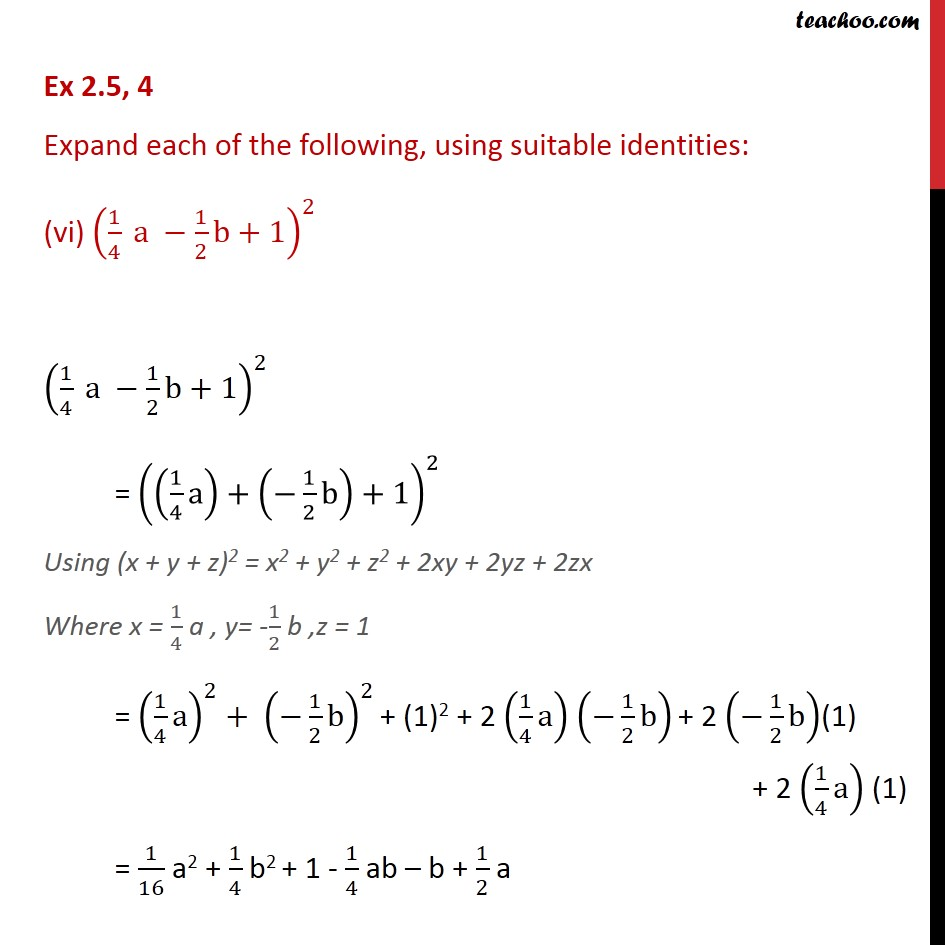1. Chapter 2 Class 9 Polynomials
2. Concept wise
3. Identity V

Transcript

Ex 2.5, 4 Expand each of the following, using suitable identities: (x + 2y + 4z)2 (x + 2y + 4z)2 Using (a + b + c)2 = a2 + b2 + c2 + 2ab + 2bc + 2ac Where a = x , b = 2y, c = 4z = x2 + (2y)2 + (4z)2 + 2(x) (2y) + 2(2y) (4z) + 2 (4z) (x) = x2 + 4y2 + 16z2 + 4xy + 16yz + 8xz Ex 2.5, 4 Expand each of the following, using suitable identities: (ii) (2x y + z)2 (2x y + z)2 = (2x + ( y) + z)2 Using (a + b + c)2 = a2 + b2 + c2 + 2ab + 2bc + 2ac Where a = 2x , b = y, c = z = (2x)2 + (-y)2 + (z)2 + 2(2x) (-y) + 2 (-y) (z) + 2 (z) (2x) = 4x2 + y2 + z2 4xy 2yz + 4xz Ex 2.5, 4 Expand each of the following, using suitable identities: (iii) (-2x + 3y + 2z)2 ( 2x + 3y + 2z)2 = (( 2x) + 3y + 2z)2 Using (a + b + c)2 = a2 + b2 + c2 + 2ab + 2bc + 2ac Where a = -2x , b = 3y, c = 2z = (-2x)2 + (3y)2 + (2z)2 + 2(-2x) (3y) + 2 (3y) (2z) + 2 (2z) (-2x) = 4x2 + 9y2 + 4z2 12xy + 12yz 8xz Ex 2.5, 4 Expand each of the following, using suitable identities: (iv) (3a 7b c)2 (3a 7b c)2 = (3a + ( 7b) + ( c) )2 Using (x + y + z)2 = x2 + y2 + z2 + 2xy + 2yz + 2zx x = 3a, y = -7b , z = -c = (3a)2 + (-7b)2 + (-c)2 + 2(3a)(-7b) + 2(-7b)(-c) + 2(-c) (3a) = 9a2 + 49b2 + c2 42ab + 14bc 6ac Ex 2.5, 4 Expand each of the following, using suitable identities: (v) (-2x + 5y 3z)2 (-2x + 5y 3z)2 = ( (-2x) + 5y + (-3z) )2 Using (a + b + c)2 = a2 + b2 + c2 + 2ab + 2bc + 2ac Where a = -2x , b = 5y, c = -3z = (-2x)2 + (5y)2 + (-3z)2 + 2(-2x) (5y) + 2 (5y) (-3z) + 2 (-3z) (-2x) = 4x2 + 25y2 + 9z2 20xy 30yz + 12xz Ex 2.5, 4 Expand each of the following, using suitable identities: (vi) (1/4 a 1/2 b+1)^2 (1/4 a 1/2 b+1)^2 = ((1/4 a)+( 1/2 b)+1)^2 Using (x + y + z)2 = x2 + y2 + z2 + 2xy + 2yz + 2zx Where x = 1/4 a , y= -1/2 b ,z = 1 = (1/4 a)^2+ ( 1/2 b)^2 + (1)2 + 2 (1/4 a) ( 1/2 b) + 2 ( 1/2 b)(1) + 2 (1/4 a) (1) = 1/16 a2 + 1/4 b2 + 1 - 1/4 ab b + 1/2 a

Identity V